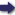(London :  Kegan Paul, Trench, Trübner & Co.,  1910.)

 Tools

## Search this bookPrev Page 204 Next``` ( 204 ) CHAPTEE LXXIX. ON TIIE YOGAS. Page 299. TiiEbE are times which the Hindus think to be most unlucky and during which they abstain from all action. They are numerous. We shall here mention them. Explanation There are two yogas regarding which all Hindus of vyali'pnta, and vaidh- agree, VIZ. :---- ■ ' (i.) The moment when sun and moon together stand on two circles, which are, as it were, seizing each other, i.e. each pair of circles, the declinations of which, on one and the same side (of either solstice), are equal. This yoga is called vyctiipdta. (2.) The moment when sun and moon stand together on two eq^v,a,l circles, i.e. each pair of circles, the de¬ clinations of which, on different sides (of either solstice), are equal. This is called vaidhritct. It is the signum of the former that in it the sum of the corrected places of sun and moon ti'epresents in any case the distance of six zodiacal signs from 0° of Aries, while it is the signum for the latter that the same sum represents the distance of twelve signs. If you compute the corrected places of sun and moon for a certain time and add them together, the sum is either of these signa, i.e. either of these two yogcts. If, however, the sum is less than the amount of the signum or larger, in that case the time of equality (i.e. the time when the sum is equal to either of the signet) is computed by means of the difference between this sum and the term in question, and by means of the ```Prev Page 204 Next